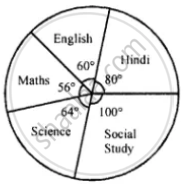# For the Data Given Above in Question Number 7, Draw a Suitable Pie-graph. - Mathematics

Chart
Sum

For the data given above in question number 7, draw a suitable pie-graph.

#### Solution

∵ 60 + 45 + 42 + 48 + 75 = 270∴ Central angle for Hindi = 60/270 xx 360^circ = 80^circ

Central angle for English = 45/270 xx 360^circ = 60^circ

Central angle for Maths = 42/270 xx 360^circ = 45^circ

Central angle for Science = 48/270 xx 360^circ = 64^circ

and Central angle for Social study = 75/270 xx 360^circ = 100^circ

Concept: Graphical Representation of Data
Is there an error in this question or solution?

#### APPEARS IN

Selina Concise Mathematics Class 8 ICSE
Chapter 22 Data Handling
Exercise 22 (B) | Q 8 | Page 253